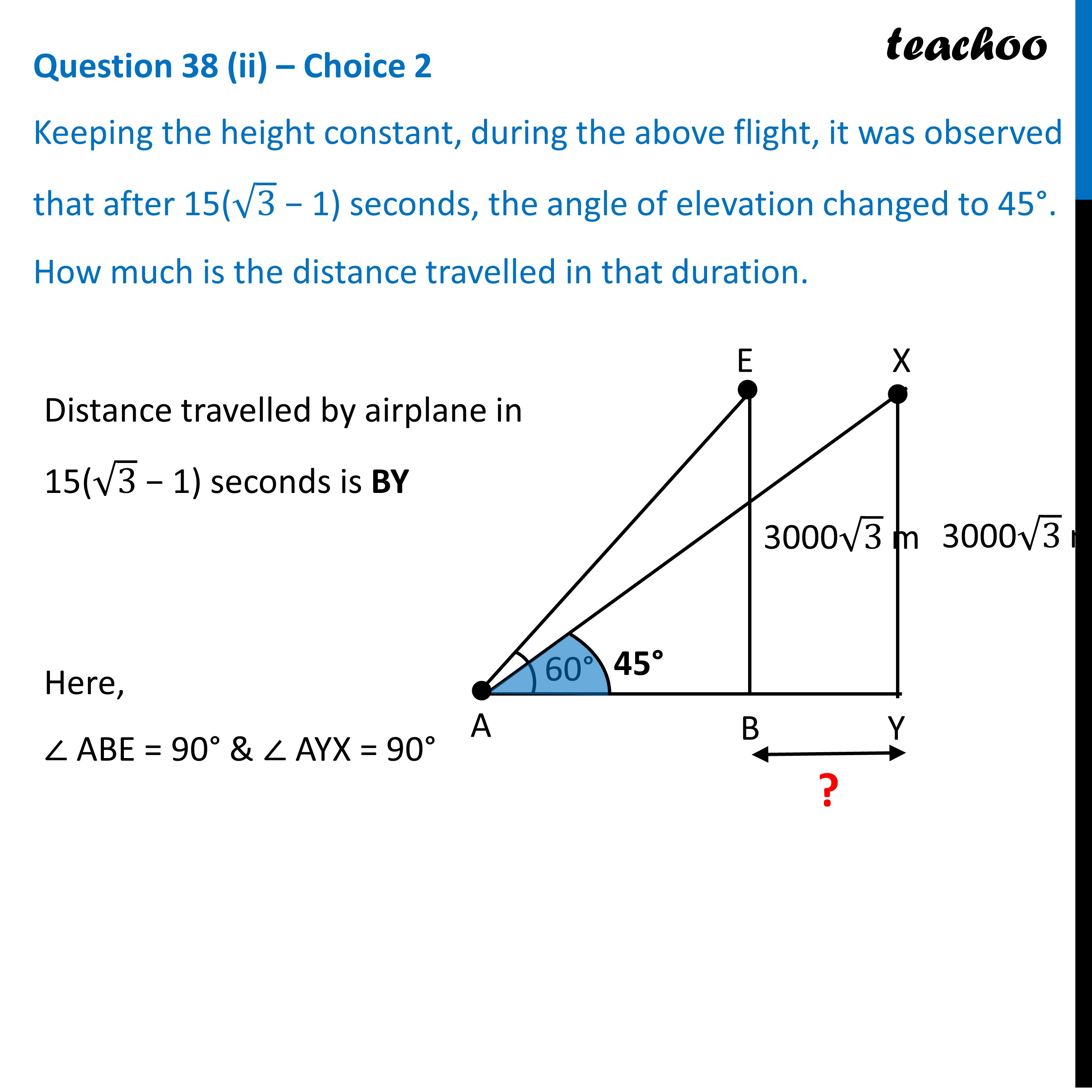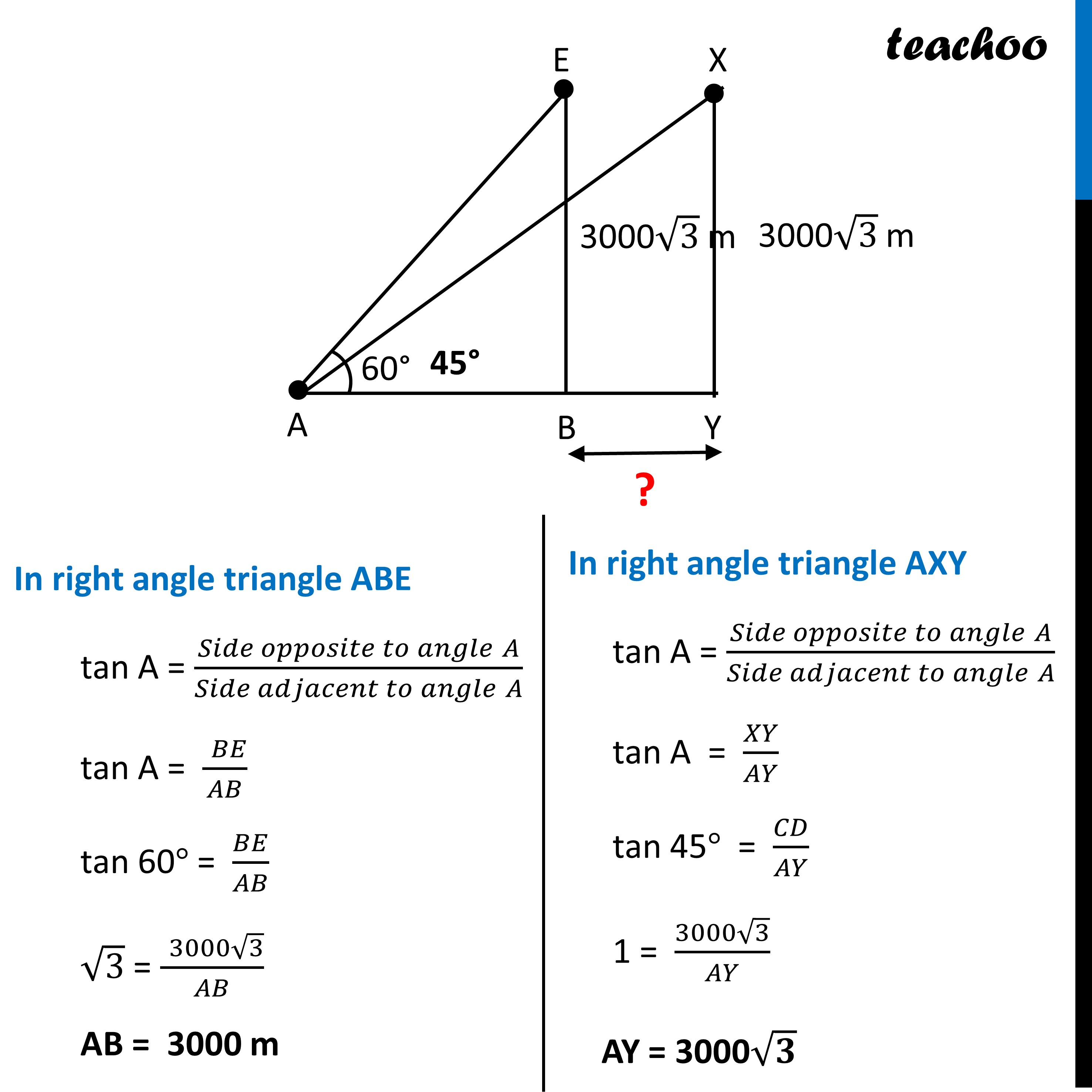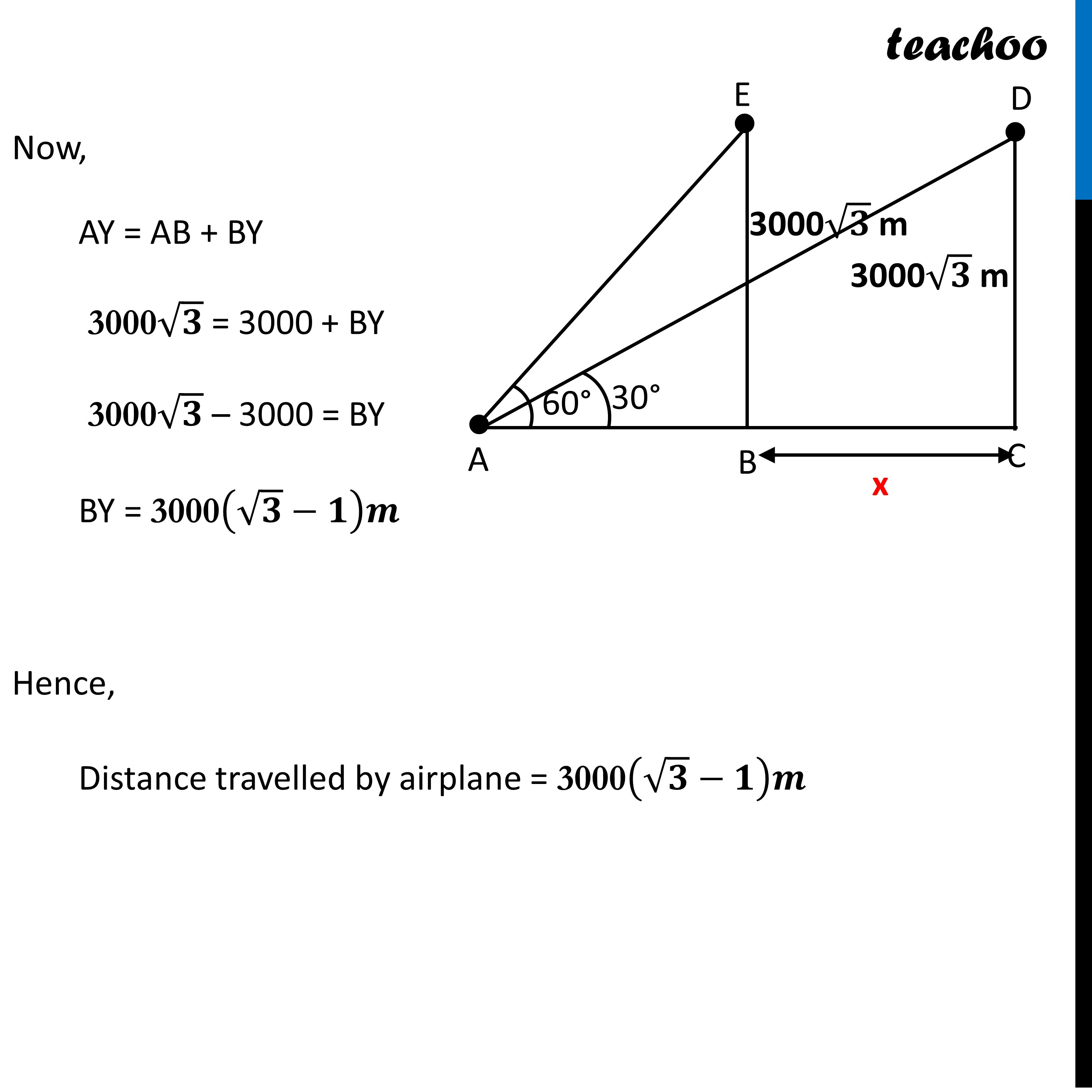CBSE Class 10 Sample Paper for 2023 Boards - Maths Standard

Class 10
Solutions of Sample Papers for Class 10 Boards

## Keeping the height constant, during the above flight, it was observed that after 15(√3-1) seconds, the angle of elevation changed to 45°. How much is the distance travelled in that duration.Learn in your speed, with individual attention - Teachoo Maths 1-on-1 Class

### Transcript

Question 38 (ii) – Choice 2 Keeping the height constant, during the above flight, it was observed that after 15(√3 − 1) seconds, the angle of elevation changed to 45°. How much is the distance travelled in that duration.Distance travelled by airplane in 15(√3 − 1) seconds is BY Here, ∠ ABE = 90° & ∠ AYX = 90° In right angle triangle ABE tan A = (𝑆𝑖𝑑𝑒 𝑜𝑝𝑝𝑜𝑠𝑖𝑡𝑒 𝑡𝑜 𝑎𝑛𝑔𝑙𝑒" " 𝐴)/(𝑆𝑖𝑑𝑒 𝑎𝑑𝑗𝑎𝑐𝑒𝑛𝑡 𝑡𝑜 𝑎𝑛𝑔𝑙𝑒" " 𝐴) tan A = (" " 𝐵𝐸)/𝐴𝐵 tan 60° = 𝐵𝐸/𝐴𝐵 √3 = (" " 3000√3)/𝐴𝐵 AB = 3000 m In right angle triangle AXY tan A = (𝑆𝑖𝑑𝑒 𝑜𝑝𝑝𝑜𝑠𝑖𝑡𝑒 𝑡𝑜 𝑎𝑛𝑔𝑙𝑒" " 𝐴)/(𝑆𝑖𝑑𝑒 𝑎𝑑𝑗𝑎𝑐𝑒𝑛𝑡 𝑡𝑜 𝑎𝑛𝑔𝑙𝑒" " 𝐴) tan A = 𝑋𝑌/𝐴𝑌 tan 45° = 𝐶𝐷/𝐴𝑌 1 = (3000√3)/𝐴𝑌 AY = 3000√𝟑 Now, AY = AB + BY 3000√𝟑 = 3000 + BY 3000√𝟑 – 3000 = BY BY = 3000(√𝟑−𝟏)𝒎 Hence, Distance travelled by airplane = 3000(√𝟑−𝟏)𝒎